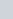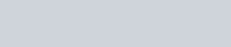What length of a solid cylinder 2 cm in diameter must
Question:

What length of a solid cylinder 2 cm in diameter must be taken to recast into a hollow cylinder of length 16 cm, external diameter 20 cm and thickness 2.5 mm?

Solution:

We are given a solid cylinder of, diameter = 2 cm

We have to recast it into a hollow cylinder of length = 16 cm

External Diameter = 20 cm and thickness = 2.5 mm=0.25 cm

We have to find the height of the solid cylinder that can be used to get a hollow cylinder of the desired dimensions.

Volume of a solid cylinder $=\pi r^{2} h$

So,

The volume of the given solid cylinder $=\pi(1)^{2} h$ (a)

Here, heighthas to be found.

Volume of a hollow cylinder $=\pi h\left(R^{2}-r^{2}\right)$

Where $R$ is the external radius and $r$ is the internal radius

External radius is given. Thickness of the hollow cylinder is also given.

So, we can find the internal radius of the hollow cylinder.

Thickness=Rr

$\Rightarrow 0.25=10-r$

$\Rightarrow r=9.75 \mathrm{~cm}$

So, the volume of the hollow cylinder $=\pi \times 16 \times(100-95.0625) \ldots \ldots$ (b)

From (a) and (b) we get,

$\pi(1)^{2} h=\pi \times 16 \times(100-95.0625)$$h=16 \times(4.9375)$

$h=79 \mathrm{~cm}$

Hence, the required height of the solid cylinder is h=79 cm